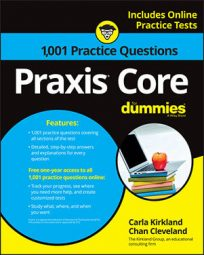##### Praxis Core: 1,001 Practice Questions For DummiesWhen you encounter a percentage problem on the Praxis Core exam, it will often be in the form of a word problem. This means you'll have to pay close attention to what you're being asked to find.

In the first practice question, you're asked to convert percentages of campers to their actual numbers, and then do some extra math to solve the problem; in the second question, you're asked to find the percent increase of a bank account.

## Practice questions

1. A group of 400 people went camping for a weekend. On Saturday, each camper engaged in one of the activities listed in the table. The table shows the percentages of campers who engaged in each activity. According to the table, which of the following statements is true?

A. Sixty-eight campers went canoeing. B. Twice as many campers went birdwatching as the number that went canoeing. C. Eighty-two campers went hiking. D. Sixteen more campers went rock climbing than canoeing. E. Twenty-one more campers went rock climbing than birdwatching.

2. Edward opened a savings account and put \$500 in it. The interest on the account is 12 percent per year. If no money is deposited in or taken out of the account, how much money will Edward have in the account exactly a year after he put in \$500?

A. \$560 B. \$60 C. \$6,000 D. \$440 E. \$620

1. The correct answer is Choice (D).

To find the number of campers who did each activity, multiply the percent for the activity by 400. You can multiply by the percents by converting the percentages to decimals.

With those numbers, you can make calculations to determine which of the answer choices is true. The only true choice is Choice (D). The number of campers who went rock climbing is 152, and the number of campers who went canoeing is 136:

152 – 136 = 16

2. The correct answer is Choice (A).

At exactly a year, the amount of interest accrued for the savings account is 12 percent of \$500:

The interest is \$60. That amount is added to the account on top of the \$500 that was already there:

500 + 60 = 560

The amount of money in the account exactly a year after Edward put \$500 in it is \$560.